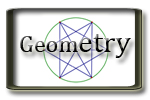G.P. Geometry Unit 7 Quadrilaterals and Other Polygons Jump to Unit 1 - 2 - 3 - 4 - 5 - 6 - 7- 8- 9 - 10 - 11 Unit 7 Textbook with Examples and extra problems. Big Ideas Video Tutorials - Lots of video examples

Lesson

Handouts/Activities

Completed Class Notes

Videos and Support Resources

7.1 Angles of Polygons
 Example 1 Example 2 Example 3 Example 4 Example 5 Example 6
7.2 Properties of Parallelograms
 Example 1 Example 2 Example 3 Example 4 Example 5
7.3 Proving that a Quadrilateral is a Parallelogram
 Example 1 Example 2 Example 3 Example 4 Example 5
7.4 Properties of Special Parallelograms
 Example 1 Example 2 Example 3 Example 4 Example 5 Example 6
7.5 Properties of Trapezoids and Kites
 Example 1 Example 2 Example 3 Example 4 Example 5 Example 6